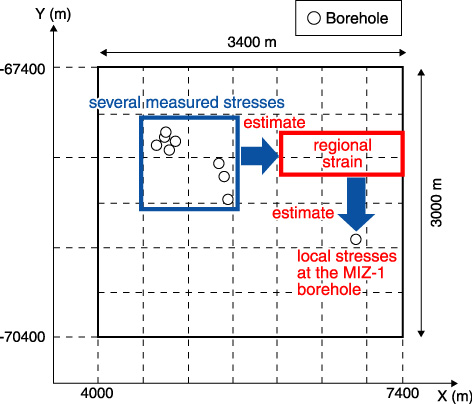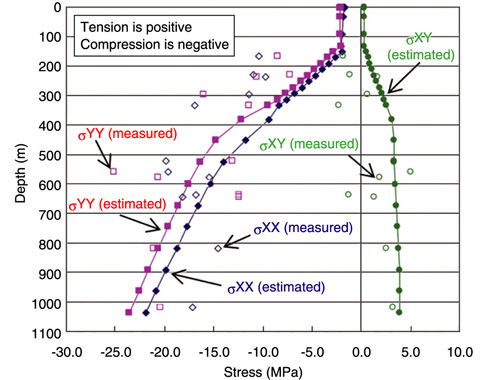# 2-7 Understanding Regional Stress in a Rock Mass

## －Estimation of Regional Stress Using Measured Stress－Fig.2-17　Analysis area and stress measurement points

The regional strain was estimated by using several measured local stresses in the area within the blue square. The local stresses at the MIZ-1 borehole were estimated by providing a displacement equivalent to the estimated regional strain around the area boundary.* σXY denotes the stress in the Y direction in the YZ plane.

Fig.2-18　Analysis area and stress measurement points

The magnitudes of the measured and calculated stresses differ slightly, but the change with depth is quite similar. In particular, the change in the slope of the curves at 600 m is evident.

For the geological disposal of high-level radioactive waste, many deep underground facilities are likely to be constructed within an area of a few square kilometers. Evaluation of the rock stress field is necessary to ensure the safe operation of the facilities and to minimize the area of the rock mass affected by excavations as well as damage to the rock mass. An understanding of the local stress is important for the evaluation of the safety of the region near the facilities. An understanding of the regional stress field is necessary to evaluate the safety in the area around the facilities. While numerous local stress measurements are needed to understand regional stress, it is difficult to obtain a large number of measurements because of cost and time constraints and labor requirements. The local stress can be obtained by using the hydraulic fracturing stress measurement technique and performing laboratory studies on drill core samples.

In this study, we propose a method for the estimation of regional stress from a limited number of local stress measurements, based on the following assumptions:
(a) The regional stress estimate depends on the boundary conditions used.
(b) The local stresses are the sum of the overburden pressure and the horizontal stress associated with plate tectonic forces.

In this study, the regional strain was used as a substitute for the regional stress because the strain is proportional to the stress in elastic analysis. The process for estimating the regional strain is described below:
(1) Model a target area on the basis of surface-based investigations and define each element on the basis of actual rock properties.
(2) Identify the reference strain and apply a displacement equivalent to it to the model boundary. Calculate the stresses corresponding to the applied displacements for the target area.
(3) Calculate the ratio of the measured stresses to the stresses calculated in (2) above by the least squares method. Estimate the regional strain by multiplying the reference strain with the ratio (Fig.2-17).

The estimated regional stress was compared with the measured stresses in the MIZ-1 borehole to evaluate the validity of the estimated regional strain. The magnitudes of the measured and calculated stresses differed slightly, but the change with depth was quite similar. In particular, the change in the slope of the curves at 600 m was evident (Fig.2-18).

| | | | |